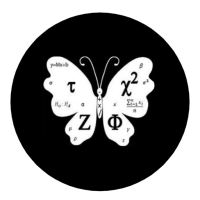## Welcome to Moore Statistics Consulting LLCEach variable type has its own probability distribution. Continuous variables follow either a Z or T distribution. Categorical Variables typically follows a frequency count distribution. That probability distribution can be a binomial, poisson, Bernoulli, or anything specific to COUNT data.

If you are dealing with a binomial distribution this is how you break it down into simpier terms:

1. The probability  of success is 1-the probability of failure. What is a success? Your event of interest. So if you are looking at disease , the probability of disease is your success rate.
2. You need to know how many successes you are talking about which is denoted by Y. So if you have 5 success then Y equals 5.
3. You need to know how large is your sample size, N.
4. The equation is: P(y=Y): N!/Y!(N-Y)! π^y (1-π)^(n-y)  where ^ denotes your exponential.

If you enjoyed this short blog, there are more to follow. -Amy